# Mobject#

Qualified name: manim.mobject.mobject.Mobject

class Mobject(color='#FFFFFF', name=None, dim=3, target=None, z_index=0)[source]#

Bases: object

Mathematical Object: base class for objects that can be displayed on screen.

There is a compatibility layer that allows for getting and setting generic attributes with get_* and set_* methods. See set() for more details.

submobjects#

The contained objects.

Type

List[Mobject]

points#

The points of the objects.

Type

numpy.ndarray

Methods

 add Add mobjects as submobjects. add_animation_override Add an animation override. add_background_rectangle Add a BackgroundRectangle as submobject. add_background_rectangle_to_family_members_with_points add_background_rectangle_to_submobjects add_n_more_submobjects add_to_back Add all passed mobjects to the back of the submobjects. add_updater Add an update function to this mobject. align_data Aligns the data of this mobject with another mobject. align_on_border Direction just needs to be a vector pointing towards side or corner in the 2d plane. align_points align_points_with_larger align_submobjects align_to Aligns mobject to another Mobject in a certain direction. animation_override_for Returns the function defining a specific animation override for this class. apply_complex_function Applies a complex function to a Mobject. apply_function apply_function_to_position apply_function_to_submobject_positions apply_matrix apply_over_attr_arrays apply_points_function_about_point apply_to_family Apply a function to self and every submobject with points recursively. arrange Sorts Mobject next to each other on screen. arrange_in_grid Arrange submobjects in a grid. arrange_submobjects Arrange the position of submobjects with a small buffer. become Edit points, colors and submobjects to be identical to another Mobject center clear_updaters Remove every updater. copy Create and return an identical copy of the Mobject including all submobjects. fade fade_to family_members_with_points flip Flips/Mirrors an mobject about its center. generate_points Initializes points and therefore the shape. generate_target get_all_points Return all points from this mobject and all submobjects. get_array_attrs get_bottom Get bottom coordinates of a box bounding the Mobject get_boundary_point get_center Get center coordinates get_center_of_mass get_color Returns the color of the Mobject get_coord Meant to generalize get_x, get_y and get_z get_corner Get corner coordinates for certain direction. get_critical_point Picture a box bounding the Mobject. get_edge_center Get edge coordinates for certain direction. get_end Returns the point, where the stroke that surrounds the Mobject ends. get_extremum_along_dim get_family get_family_updaters get_group_class get_image get_left Get left coordinates of a box bounding the Mobject get_merged_array Return all of a given attribute from this mobject and all submobjects. get_midpoint Get coordinates of the middle of the path that forms the Mobject. get_mobject_type_class Return the base class of this mobject type. get_nadir Get nadir (opposite the zenith) coordinates of a box bounding a 3D Mobject. get_num_points get_pieces get_point_mobject The simplest Mobject to be transformed to or from self. get_points_defining_boundary get_right Get right coordinates of a box bounding the Mobject get_start Returns the point, where the stroke that surrounds the Mobject starts. get_start_and_end Returns starting and ending point of a stroke as a tuple. get_time_based_updaters Return all updaters using the dt parameter. get_top Get top coordinates of a box bounding the Mobject get_updaters Return all updaters. get_x Returns x coordinate of the center of the Mobject as float get_y Returns y coordinate of the center of the Mobject as float get_z Returns z coordinate of the center of the Mobject as float get_z_index_reference_point get_zenith Get zenith coordinates of a box bounding a 3D Mobject. has_no_points Check if Mobject does not contains points. has_points Check if Mobject contains points. has_time_based_updater Test if self has a time based updater. init_colors Initializes the colors. insert Inserts a mobject at a specific position into self.submobjects interpolate Turns this Mobject into an interpolation between mobject1 and mobject2. interpolate_color invert Inverts the list of submobjects. is_off_screen length_over_dim Measure the length of an Mobject in a certain direction. match_color Match the color with the color of another Mobject. match_coord Match the coordinates with the coordinates of another Mobject. match_depth Match the depth with the depth of another Mobject. match_dim_size Match the specified dimension with the dimension of another Mobject. match_height Match the height with the height of another Mobject. match_points Edit points, positions, and submobjects to be identical to another Mobject, while keeping the style unchanged. match_updaters Match the updaters of the given mobject. match_width Match the width with the width of another Mobject. match_x Match x coord. match_y Match y coord. match_z Match z coord. move_to Move center of the Mobject to certain coordinate. next_to Move this Mobject next to another's Mobject or coordinate. nonempty_submobjects null_point_align If a Mobject with points is being aligned to one without, treat both as groups, and push the one with points into its own submobjects list. point_from_proportion pose_at_angle proportion_from_point push_self_into_submobjects put_start_and_end_on reduce_across_dimension Find the min or max value from a dimension across all points in this and submobjects. remove Remove submobjects. remove_updater Remove an updater. repeat This can make transition animations nicer repeat_submobject replace rescale_to_fit reset_points Sets points to be an empty array. restore Restores the state that was previously saved with save_state(). resume_updating Enable updating from updaters and animations. reverse_points rotate Rotates the Mobject about a certain point. rotate_about_origin Rotates the Mobject about the ORIGIN, which is at [0,0,0]. save_image Saves an image of only this Mobject at its position to a png file. save_state Save the current state (position, color & size). scale Scale the size by a factor. scale_to_fit_depth Scales the Mobject to fit a depth while keeping width/height proportional. scale_to_fit_height Scales the Mobject to fit a height while keeping width/depth proportional. scale_to_fit_width Scales the Mobject to fit a width while keeping height/depth proportional. set Sets attributes. set_color Condition is function which takes in one arguments, (x, y, z). set_color_by_gradient set_colors_by_radial_gradient set_coord set_default Sets the default values of keyword arguments. set_submobject_colors_by_gradient set_submobject_colors_by_radial_gradient set_x Set x value of the center of the Mobject (int or float) set_y Set y value of the center of the Mobject (int or float) set_z Set z value of the center of the Mobject (int or float) set_z_index Sets the Mobject's z_index to the value specified in z_index_value. set_z_index_by_z_coordinate Sets the Mobject's z coordinate to the value of z_index. shift Shift by the given vectors. shift_onto_screen show shuffle Shuffles the list of submobjects. shuffle_submobjects Shuffles the order of submobjects sort Sorts the list of submobjects by a function defined by submob_func. sort_submobjects Sort the submobjects space_out_submobjects split stretch stretch_about_point stretch_to_fit_depth Stretches the Mobject to fit a depth, not keeping width/height proportional. stretch_to_fit_height Stretches the Mobject to fit a height, not keeping width/depth proportional. stretch_to_fit_width Stretches the Mobject to fit a width, not keeping height/depth proportional. surround suspend_updating Disable updating from updaters and animations. throw_error_if_no_points to_corner to_edge to_original_color update Apply all updaters. wag

Attributes

 animate Used to animate the application of any method of self. animation_overrides depth The depth of the mobject. height The height of the mobject. width The width of the mobject.

The mobjects are added to submobjects.

Subclasses of mobject may implement + and += dunder methods.

Parameters

mobjects (Mobject) – The mobjects to add.

Returns

self

Return type

Mobject

Raises
• ValueError – When a mobject tries to add itself.

• TypeError – When trying to add an object that is not an instance of Mobject.

Notes

A mobject cannot contain itself, and it cannot contain a submobject more than once. If the parent mobject is displayed, the newly-added submobjects will also be displayed (i.e. they are automatically added to the parent Scene).

Examples

>>> outer = Mobject()
>>> inner = Mobject()


>>> outer = outer.add(inner)
>>> len(outer.submobjects)
1


Adding an object to itself raises an error:

>>> outer.add(outer)
Traceback (most recent call last):
...
ValueError: Mobject cannot contain self


A given mobject cannot be added as a submobject twice to some parent:

>>> parent = Mobject(name="parent")
>>> child = Mobject(name="child")
[...] WARNING  ...
parent
>>> parent.submobjects
[child]


This does not apply to subclasses.

Parameters
• animation_class (type[Animation]) – The animation type to be overridden

• override_func (Callable[[Mobject, ...], Animation]) – The function returning an animation replacing the default animation. It gets passed the parameters given to the animnation constructor.

Raises

MultiAnimationOverrideException – If the overridden animation was already overridden.

The BackgroundRectangle is added behind other submobjects.

This can be used to increase the mobjects visibility in front of a noisy background.

Parameters
• color (Colors | None) – The color of the BackgroundRectangle

• opacity (float) – The opacity of the BackgroundRectangle

• kwargs – Additional keyword arguments passed to the BackgroundRectangle constructor

Returns

self

Return type

Mobject

Add all passed mobjects to the back of the submobjects.

If submobjects already contains the given mobjects, they just get moved to the back instead.

Parameters

mobjects (Mobject) – The mobjects to add.

Returns

self

Return type

Mobject

Note

Technically, this is done by adding (or moving) the mobjects to the head of submobjects. The head of this list is rendered first, which places the corresponding mobjects behind the subsequent list members.

Raises
• ValueError – When a mobject tries to add itself.

• TypeError – When trying to add an object that is not an instance of Mobject.

Parameters

mobjects (Mobject) –

Notes

A mobject cannot contain itself, and it cannot contain a submobject more than once. If the parent mobject is displayed, the newly-added submobjects will also be displayed (i.e. they are automatically added to the parent Scene).

Add an update function to this mobject.

Update functions, or updaters in short, are functions that are applied to the Mobject in every frame.

Parameters
• update_function (Updater) – The update function to be added. Whenever update() is called, this update function gets called using self as the first parameter. The updater can have a second parameter dt. If it uses this parameter, it gets called using a second value dt, usually representing the time in seconds since the last call of update().

• index (int | None) – The index at which the new updater should be added in self.updaters. In case index is None the updater will be added at the end.

• call_updater (bool) – Whether or not to call the updater initially. If True, the updater will be called using dt=0.

Returns

self

Return type

Mobject

Examples

Example: NextToUpdater

from manim import *

class NextToUpdater(Scene):
def construct(self):
def dot_position(mobject):
mobject.set_value(dot.get_center())
mobject.next_to(dot)

dot = Dot(RIGHT*3)
label = DecimalNumber()



Example: DtUpdater

from manim import *

class DtUpdater(Scene):
def construct(self):
line = Square()

#Let the line rotate 90° per second
self.wait(2)

align_data(mobject, skip_point_alignment=False)[source]#

Aligns the data of this mobject with another mobject.

Afterwards, the two mobjects will have the same number of submobjects (see align_submobjects()), the same parent structure (see null_point_align()). If skip_point_alignment is false, they will also have the same number of points (see align_points()).

Parameters
• mobject (Mobject) – The other mobject this mobject should be aligned to.

• skip_point_alignment (bool) – Controls whether or not the computationally expensive point alignment is skipped (default: False).

align_on_border(direction, buff=0.5)[source]#

Direction just needs to be a vector pointing towards side or corner in the 2d plane.

align_to(mobject_or_point, direction=array([0., 0., 0.]))[source]#

Aligns mobject to another Mobject in a certain direction.

Examples: mob1.align_to(mob2, UP) moves mob1 vertically so that its top edge lines ups with mob2’s top edge.

Parameters

mobject_or_point (Mobject | np.ndarray | list) –

property animate: _AnimationBuilder | T#

Used to animate the application of any method of self.

Any method called on animate is converted to an animation of applying that method on the mobject itself.

For example, square.set_fill(WHITE) sets the fill color of a square, while square.animate.set_fill(WHITE) animates this action.

Multiple methods can be put in a single animation once via chaining:

self.play(my_mobject.animate.shift(RIGHT).rotate(PI))


Warning

Passing multiple animations for the same Mobject in one call to play() is discouraged and will most likely not work properly. Instead of writing an animation like

self.play(my_mobject.animate.shift(RIGHT), my_mobject.animate.rotate(PI))


make use of method chaining.

Keyword arguments that can be passed to Scene.play() can be passed directly after accessing .animate, like so:

self.play(my_mobject.animate(rate_func=linear).shift(RIGHT))


This is especially useful when animating simultaneous .animate calls that you want to behave differently:

self.play(
mobject1.animate(run_time=2).rotate(PI),
mobject2.animate(rate_func=there_and_back).shift(RIGHT),
)


Examples

Example: AnimateExample

from manim import *

class AnimateExample(Scene):
def construct(self):
s = Square()
self.play(Create(s))
self.play(s.animate.shift(RIGHT))
self.play(s.animate.scale(2))
self.play(s.animate.rotate(PI / 2))
self.play(Uncreate(s))


Example: AnimateChainExample

from manim import *

class AnimateChainExample(Scene):
def construct(self):
s = Square()
self.play(Create(s))
self.play(s.animate.shift(RIGHT).scale(2).rotate(PI / 2))
self.play(Uncreate(s))


Example: AnimateWithArgsExample

from manim import *

class AnimateWithArgsExample(Scene):
def construct(self):
s = Square()
c = Circle()

VGroup(s, c).arrange(RIGHT, buff=2)

self.play(
s.animate(run_time=2).rotate(PI / 2),
c.animate(rate_func=there_and_back).shift(RIGHT),
)


Warning

.animate

will interpolate the Mobject between its points prior to .animate and its points after applying .animate to it. This may result in unexpected behavior when attempting to interpolate along paths, or rotations. If you want animations to consider the points between, consider using ValueTracker with updaters instead.

classmethod animation_override_for(animation_class)[source]#

Returns the function defining a specific animation override for this class.

Parameters

animation_class (type[Animation]) – The animation class for which the override function should be returned.

Returns

The function returning the override animation or None if no such animation override is defined.

Return type

Optional[Callable[[Mobject, …], Animation]]

apply_complex_function(function, **kwargs)[source]#

Applies a complex function to a Mobject. The x and y coordinates correspond to the real and imaginary parts respectively.

Example

Example: ApplyFuncExample

from manim import *

class ApplyFuncExample(Scene):
def construct(self):
circ = Circle().scale(1.5)
circ_ref = circ.copy()
circ.apply_complex_function(
lambda x: np.exp(x*1j)
)
t = ValueTracker(0)
lambda x: x.become(circ_ref.copy().apply_complex_function(
lambda x: np.exp(x+t.get_value()*1j)
)).set_color(BLUE)
)
self.play(TransformFromCopy(circ_ref, circ))
self.play(t.animate.set_value(TAU), run_time=3)

apply_to_family(func)[source]#

Apply a function to self and every submobject with points recursively.

Parameters

func (Callable[[Mobject], None]) – The function to apply to each mobject. func gets passed the respective (sub)mobject as parameter.

Returns

self

Return type

Mobject

family_members_with_points()

arrange(direction=array([1., 0., 0.]), buff=0.25, center=True, **kwargs)[source]#

Sorts Mobject next to each other on screen.

Examples

Example: Example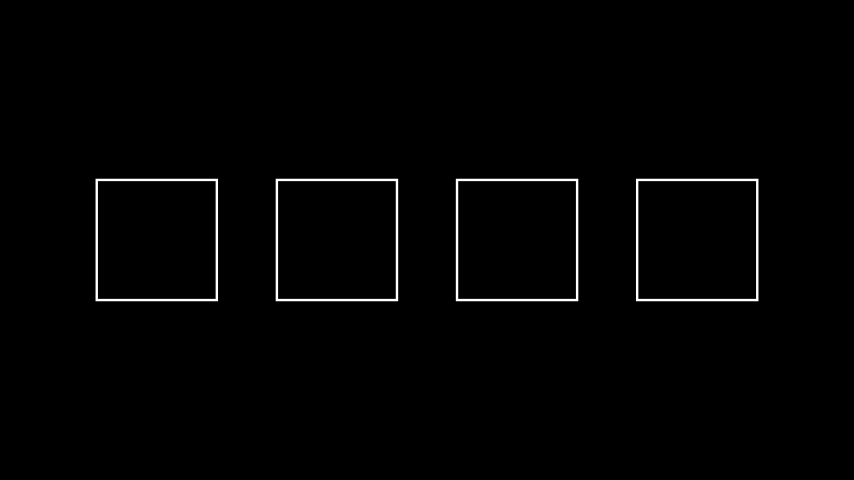from manim import *

class Example(Scene):
def construct(self):
s1 = Square()
s2 = Square()
s3 = Square()
s4 = Square()
x = VGroup(s1, s2, s3, s4).set_x(0).arrange(buff=1.0)

Parameters

direction (Sequence[float]) –

arrange_in_grid(rows=None, cols=None, buff=0.25, cell_alignment=array([0., 0., 0.]), row_alignments=None, col_alignments=None, row_heights=None, col_widths=None, flow_order='rd', **kwargs)[source]#

Arrange submobjects in a grid.

Parameters
• rows (int | None) – The number of rows in the grid.

• cols (int | None) – The number of columns in the grid.

• buff (float | tuple[float, float]) – The gap between grid cells. To specify a different buffer in the horizontal and vertical directions, a tuple of two values can be given - (row, col).

• cell_alignment (np.ndarray) – The way each submobject is aligned in its grid cell.

• row_alignments (str | None) – The vertical alignment for each row (top to bottom). Accepts the following characters: "u" - up, "c" - center, "d" - down.

• col_alignments (str | None) – The horizontal alignment for each column (left to right). Accepts the following characters "l" - left, "c" - center, "r" - right.

• row_heights (Iterable[float | None] | None) – Defines a list of heights for certain rows (top to bottom). If the list contains None, the corresponding row will fit its height automatically based on the highest element in that row.

• col_widths (Iterable[float | None] | None) – Defines a list of widths for certain columns (left to right). If the list contains None, the corresponding column will fit its width automatically based on the widest element in that column.

• flow_order (str) – The order in which submobjects fill the grid. Can be one of the following values: “rd”, “dr”, “ld”, “dl”, “ru”, “ur”, “lu”, “ul”. (“rd” -> fill rightwards then downwards)

Returns

self

Return type

Mobject

Raises
• ValueError – If rows and cols are too small to fit all submobjects.

• ValueError – If cols, col_alignments and col_widths or rows, row_alignments and row_heights have mismatching sizes.

Notes

If only one of cols and rows is set implicitly, the other one will be chosen big enough to fit all submobjects. If neither is set, they will be chosen to be about the same, tending towards cols > rows (simply because videos are wider than they are high).

If both cell_alignment and row_alignments / col_alignments are defined, the latter has higher priority.

Examples

Example: ExampleBoxes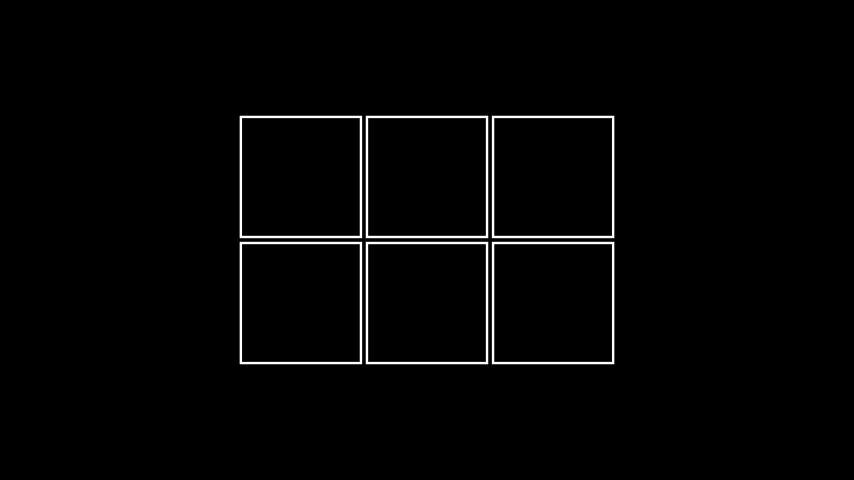from manim import *

class ExampleBoxes(Scene):
def construct(self):
boxes=VGroup(*[Square() for s in range(0,6)])
boxes.arrange_in_grid(rows=2, buff=0.1)


Example: ArrangeInGrid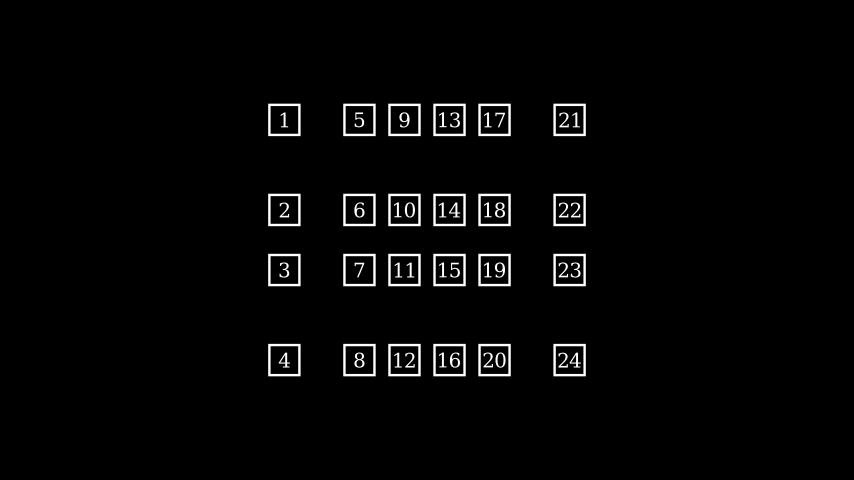from manim import *

class ArrangeInGrid(Scene):
def construct(self):
boxes = VGroup(*[
for i in range(24)
])

boxes.arrange_in_grid(
buff=(0.25,0.5),
col_alignments="lccccr",
row_alignments="uccd",
col_widths=[1, *[None]*4, 1],
row_heights=[1, None, None, 1],
flow_order="dr"
)

arrange_submobjects(*args, **kwargs)[source]#

Arrange the position of submobjects with a small buffer.

Examples

Example: ArrangeSumobjectsExample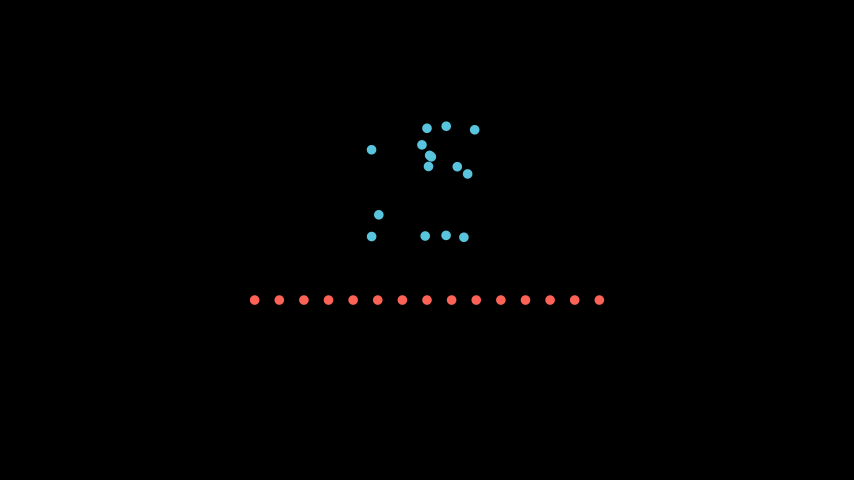from manim import *

class ArrangeSumobjectsExample(Scene):
def construct(self):
s= VGroup(*[Dot().shift(i*0.1*RIGHT*np.random.uniform(-1,1)+UP*np.random.uniform(-1,1)) for i in range(0,15)])
s.shift(UP).set_color(BLUE)
s2= s.copy().set_color(RED)
s2.arrange_submobjects()
s2.shift(DOWN)

become(mobject, copy_submobjects=True, match_height=False, match_width=False, match_depth=False, match_center=False, stretch=False)[source]#

Edit points, colors and submobjects to be identical to another Mobject

Note

If both match_height and match_width are True then the transformed Mobject will match the height first and then the width

Parameters
• match_height (bool) – If True, then the transformed Mobject will match the height of the original

• match_width (bool) – If True, then the transformed Mobject will match the width of the original

• match_depth (bool) – If True, then the transformed Mobject will match the depth of the original

• match_center (bool) – If True, then the transformed Mobject will match the center of the original

• stretch (bool) – If True, then the transformed Mobject will stretch to fit the proportions of the original

• mobject (Mobject) –

• copy_submobjects (bool) –

Examples

Example: BecomeScene

from manim import *

class BecomeScene(Scene):
def construct(self):
circ = Circle(fill_color=RED, fill_opacity=0.8)
square = Square(fill_color=BLUE, fill_opacity=0.2)
self.wait(0.5)
circ.become(square)
self.wait(0.5)

clear_updaters(recursive=True)[source]#

Remove every updater.

Parameters

recursive (bool) – Whether to recursively call clear_updaters on all submobjects.

Returns

self

Return type

Mobject

copy()[source]#

Create and return an identical copy of the Mobject including all submobjects.

Returns

The copy.

Return type

Mobject

Parameters

self (T) –

Note

The clone is initially not visible in the Scene, even if the original was.

property depth#

The depth of the mobject.

Return type

float

flip(axis=array([0., 1., 0.]), **kwargs)[source]#

Flips/Mirrors an mobject about its center.

Examples

Example: FlipExample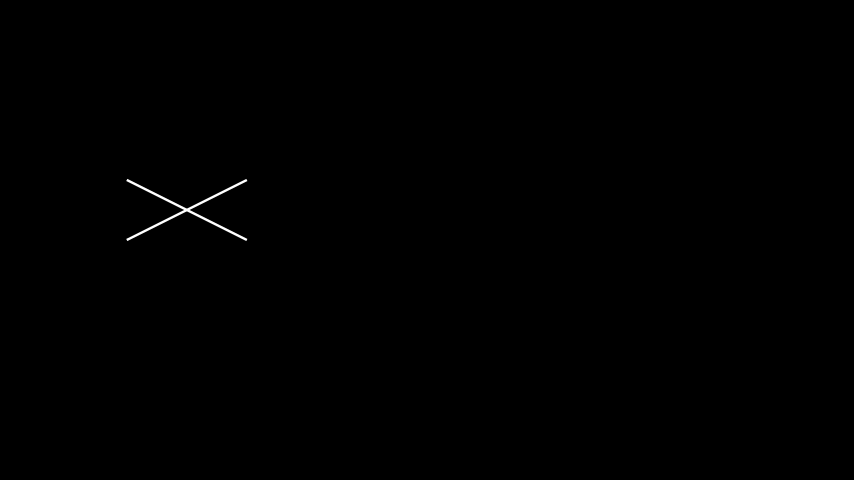from manim import *

class FlipExample(Scene):
def construct(self):
s= Line(LEFT, RIGHT+UP).shift(4*LEFT)
s2= s.copy().flip()

generate_points()[source]#

Initializes points and therefore the shape.

Gets called upon creation. This is an empty method that can be implemented by subclasses.

get_all_points()[source]#

Return all points from this mobject and all submobjects.

May contain duplicates; the order is in a depth-first (pre-order) traversal of the submobjects.

Return type

ndarray

get_bottom()[source]#

Get bottom coordinates of a box bounding the Mobject

Return type

ndarray

get_center()[source]#

Get center coordinates

Return type

ndarray

get_color()[source]#

Returns the color of the Mobject

get_coord(dim, direction=array([0., 0., 0.]))[source]#

Meant to generalize get_x, get_y and get_z

get_corner(direction)[source]#

Get corner coordinates for certain direction.

Return type

ndarray

get_critical_point(direction)[source]#

Picture a box bounding the Mobject. Such a box has 9 ‘critical points’: 4 corners, 4 edge center, the center. This returns one of them, along the given direction.

sample = Arc(start_angle=PI/7, angle = PI/5)

# These are all equivalent
max_y_1 = sample.get_top()
max_y_2 = sample.get_critical_point(UP)
max_y_3 = sample.get_extremum_along_dim(dim=1, key=1)

get_edge_center(direction)[source]#

Get edge coordinates for certain direction.

Return type

ndarray

get_end()[source]#

Returns the point, where the stroke that surrounds the Mobject ends.

get_left()[source]#

Get left coordinates of a box bounding the Mobject

Return type

ndarray

get_merged_array(array_attr)[source]#

Return all of a given attribute from this mobject and all submobjects.

May contain duplicates; the order is in a depth-first (pre-order) traversal of the submobjects.

Return type

ndarray

get_midpoint()[source]#

Get coordinates of the middle of the path that forms the Mobject.

Examples

Example: AngleMidPoint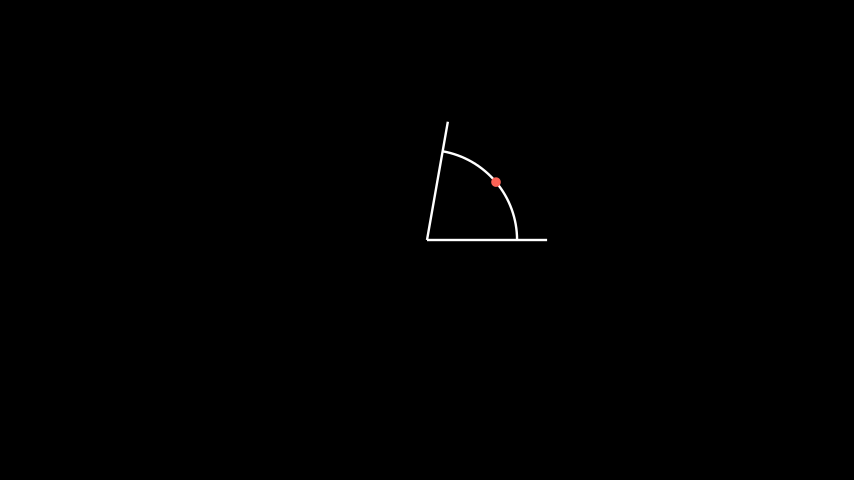from manim import *

class AngleMidPoint(Scene):
def construct(self):
line1 = Line(ORIGIN, 2*RIGHT)

a = Angle(line1, line2, radius=1.5, other_angle=False)
d = Dot(a.get_midpoint()).set_color(RED)

self.wait()

Return type

ndarray

static get_mobject_type_class()[source]#

Return the base class of this mobject type.

Get nadir (opposite the zenith) coordinates of a box bounding a 3D Mobject.

Return type

ndarray

get_point_mobject(center=None)[source]#

The simplest Mobject to be transformed to or from self. Should by a point of the appropriate type

get_right()[source]#

Get right coordinates of a box bounding the Mobject

Return type

ndarray

get_start()[source]#

Returns the point, where the stroke that surrounds the Mobject starts.

get_start_and_end()[source]#

Returns starting and ending point of a stroke as a tuple.

get_time_based_updaters()[source]#

Return all updaters using the dt parameter.

The updaters use this parameter as the input for difference in time.

Returns

The list of time based updaters.

Return type

List[Callable]

get_top()[source]#

Get top coordinates of a box bounding the Mobject

Return type

ndarray

get_updaters()[source]#

Return all updaters.

Returns

The list of updaters.

Return type

List[Callable]

get_x(direction=array([0., 0., 0.]))[source]#

Returns x coordinate of the center of the Mobject as float

Return type

float64

get_y(direction=array([0., 0., 0.]))[source]#

Returns y coordinate of the center of the Mobject as float

Return type

float64

get_z(direction=array([0., 0., 0.]))[source]#

Returns z coordinate of the center of the Mobject as float

Return type

float64

get_zenith()[source]#

Get zenith coordinates of a box bounding a 3D Mobject.

Return type

ndarray

has_no_points()[source]#

Check if Mobject does not contains points.

Return type

bool

has_points()[source]#

Check if Mobject contains points.

Return type

bool

has_time_based_updater()[source]#

Test if self has a time based updater.

Returns

classTrue if at least one updater uses the dt parameter, False otherwise.

Return type

bool

property height#

The height of the mobject.

Return type

float

Examples

Example: HeightExample

from manim import *

class HeightExample(Scene):
def construct(self):
decimal = DecimalNumber().to_edge(UP)
rect = Rectangle(color=BLUE)
rect_copy = rect.copy().set_stroke(GRAY, opacity=0.5)

self.play(rect.animate.set(height=5))
self.wait()

init_colors()[source]#

Initializes the colors.

Gets called upon creation. This is an empty method that can be implemented by subclasses.

insert(index, mobject)[source]#

Inserts a mobject at a specific position into self.submobjects

Effectively just calls self.submobjects.insert(index, mobject), where self.submobjects is a list.

Highly adapted from Mobject.add.

Parameters
• index (int) – The index at which

• mobject (Mobject) – The mobject to be inserted.

interpolate(mobject1, mobject2, alpha, path_func=<function interpolate>)[source]#

Turns this Mobject into an interpolation between mobject1 and mobject2.

Examples

Example: DotInterpolationfrom manim import *

class DotInterpolation(Scene):
def construct(self):
dotR = Dot(color=DARK_GREY)
dotR.shift(2 * RIGHT)
dotL = Dot(color=WHITE)
dotL.shift(2 * LEFT)

dotMiddle = VMobject().interpolate(dotL, dotR, alpha=0.3)


invert(recursive=False)[source]#

Inverts the list of submobjects.

Parameters

recursive – If True, all submobject lists of this mobject’s family are inverted.

Examples

Example: InvertSumobjectsExample

from manim import *

class InvertSumobjectsExample(Scene):
def construct(self):
s = VGroup(*[Dot().shift(i*0.1*RIGHT) for i in range(-20,20)])
s2 = s.copy()
s2.invert()
s2.shift(DOWN)
self.play(Write(s), Write(s2))

length_over_dim(dim)[source]#

Measure the length of an Mobject in a certain direction.

match_color(mobject)[source]#

Match the color with the color of another Mobject.

Parameters

mobject (Mobject) –

match_coord(mobject, dim, direction=array([0., 0., 0.]))[source]#

Match the coordinates with the coordinates of another Mobject.

Parameters

mobject (Mobject) –

match_depth(mobject, **kwargs)[source]#

Match the depth with the depth of another Mobject.

Parameters

mobject (Mobject) –

match_dim_size(mobject, dim, **kwargs)[source]#

Match the specified dimension with the dimension of another Mobject.

Parameters

mobject (Mobject) –

match_height(mobject, **kwargs)[source]#

Match the height with the height of another Mobject.

Parameters

mobject (Mobject) –

match_points(mobject, copy_submobjects=True)[source]#

Edit points, positions, and submobjects to be identical to another Mobject, while keeping the style unchanged.

Examples

Example: MatchPointsScene

from manim import *

class MatchPointsScene(Scene):
def construct(self):
circ = Circle(fill_color=RED, fill_opacity=0.8)
square = Square(fill_color=BLUE, fill_opacity=0.2)
self.wait(0.5)
self.play(circ.animate.match_points(square))
self.wait(0.5)

Parameters
• mobject (Mobject) –

• copy_submobjects (bool) –

match_updaters(mobject)[source]#

Match the updaters of the given mobject.

Parameters

mobject (Mobject) – The mobject whose updaters get matched.

Returns

self

Return type

Mobject

Note

All updaters from submobjects are removed, but only updaters of the given mobject are matched, not those of it’s submobjects.

match_width(mobject, **kwargs)[source]#

Match the width with the width of another Mobject.

Parameters

mobject (Mobject) –

match_x(mobject, direction=array([0., 0., 0.]))[source]#

Match x coord. to the x coord. of another Mobject.

Parameters

mobject (Mobject) –

match_y(mobject, direction=array([0., 0., 0.]))[source]#

Match y coord. to the x coord. of another Mobject.

Parameters

mobject (Mobject) –

match_z(mobject, direction=array([0., 0., 0.]))[source]#

Match z coord. to the x coord. of another Mobject.

Parameters

mobject (Mobject) –

move_to(point_or_mobject, aligned_edge=array([0., 0., 0.]), coor_mask=array([1, 1, 1]))[source]#

Move center of the Mobject to certain coordinate.

next_to(mobject_or_point, direction=array([1., 0., 0.]), buff=0.25, aligned_edge=array([0., 0., 0.]), submobject_to_align=None, index_of_submobject_to_align=None, coor_mask=array([1, 1, 1]))[source]#

Move this Mobject next to another’s Mobject or coordinate.

Examples

Example: GeometricShapes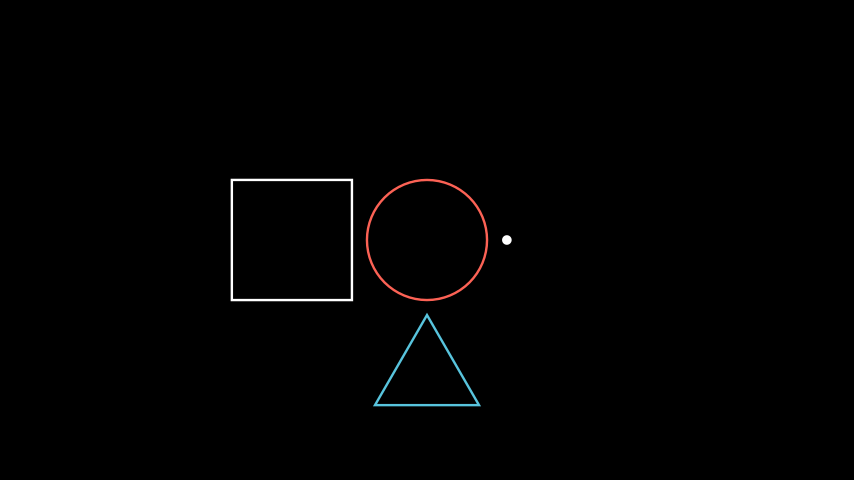from manim import *

class GeometricShapes(Scene):
def construct(self):
d = Dot()
c = Circle()
s = Square()
t = Triangle()
d.next_to(c, RIGHT)
s.next_to(c, LEFT)
t.next_to(c, DOWN)

null_point_align(mobject)[source]#

If a Mobject with points is being aligned to one without, treat both as groups, and push the one with points into its own submobjects list.

Returns

self

Return type

Mobject

Parameters

mobject (Mobject) –

reduce_across_dimension(reduce_func, dim)[source]#

Find the min or max value from a dimension across all points in this and submobjects.

Parameters

dim (int) –

Return type

float

remove(*mobjects)[source]#

Remove submobjects.

The mobjects are removed from submobjects, if they exist.

Subclasses of mobject may implement - and -= dunder methods.

Parameters

mobjects (Mobject) – The mobjects to remove.

Returns

self

Return type

Mobject

remove_updater(update_function)[source]#

Remove an updater.

If the same updater is applied multiple times, every instance gets removed.

Parameters

update_function (Union[Callable[[Mobject], None], Callable[[Mobject, float], None]]) – The update function to be removed.

Returns

self

Return type

Mobject

repeat(count)[source]#

This can make transition animations nicer

Parameters

count (int) –

reset_points()[source]#

Sets points to be an empty array.

restore()[source]#

Restores the state that was previously saved with save_state().

resume_updating(recursive=True)[source]#

Enable updating from updaters and animations.

Parameters

recursive (bool) – Whether to recursively enable updating on all submobjects.

Returns

self

Return type

Mobject

rotate(angle, axis=array([0., 0., 1.]), about_point=None, **kwargs)[source]#

Rotates the Mobject about a certain point.

Parameters

Rotates the Mobject about the ORIGIN, which is at [0,0,0].

save_image(name=None)[source]#

Saves an image of only this Mobject at its position to a png file.

save_state()[source]#

Save the current state (position, color & size). Can be restored with restore().

scale(scale_factor, **kwargs)[source]#

Scale the size by a factor.

Default behavior is to scale about the center of the mobject.

Parameters
• scale_factor (float) – The scaling factor $$\alpha$$. If $$0 < |\alpha| < 1$$, the mobject will shrink, and for $$|\alpha| > 1$$ it will grow. Furthermore, if $$\alpha < 0$$, the mobject is also flipped.

• kwargs – Additional keyword arguments passed to apply_points_function_about_point().

Returns

self

Return type

Mobject

Examples

Example: MobjectScaleExample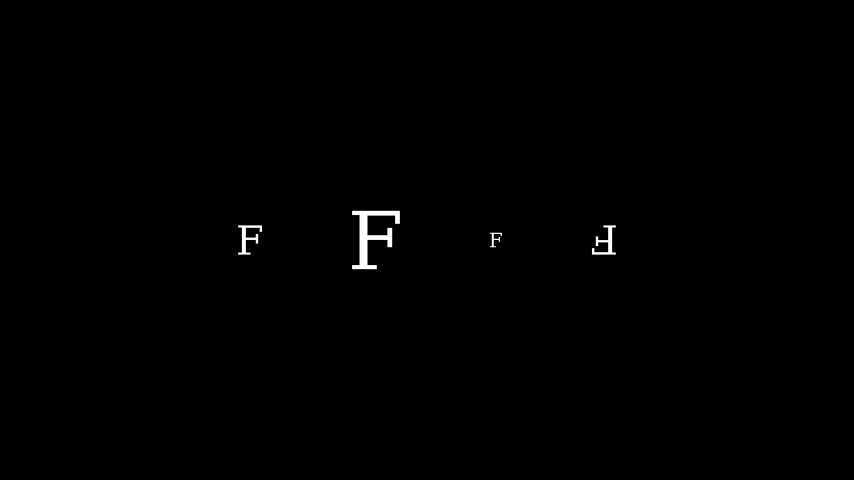from manim import *

class MobjectScaleExample(Scene):
def construct(self):
f1 = Text("F")
f2 = Text("F").scale(2)
f3 = Text("F").scale(0.5)
f4 = Text("F").scale(-1)

vgroup = VGroup(f1, f2, f3, f4).arrange(6 * RIGHT)

scale_to_fit_depth(depth, **kwargs)[source]#

Scales the Mobject to fit a depth while keeping width/height proportional.

scale_to_fit_height(height, **kwargs)[source]#

Scales the Mobject to fit a height while keeping width/depth proportional.

Returns

self

Return type

Mobject

Examples

>>> from manim import *
>>> sq = Square()
>>> sq.width
2.0
>>> sq.scale_to_fit_height(5)
Square
>>> sq.height
5.0
>>> sq.width
5.0

scale_to_fit_width(width, **kwargs)[source]#

Scales the Mobject to fit a width while keeping height/depth proportional.

Returns

self

Return type

Mobject

Examples

>>> from manim import *
>>> sq = Square()
>>> sq.height
2.0
>>> sq.scale_to_fit_width(5)
Square
>>> sq.width
5.0
>>> sq.height
5.0

set(**kwargs)[source]#

Sets attributes.

I.e. my_mobject.set(foo=1) applies my_mobject.foo = 1.

This is a convenience to be used along with animate to animate setting attributes.

In addition to this method, there is a compatibility layer that allows get_* and set_* methods to get and set generic attributes. For instance:

>>> mob = Mobject()
>>> mob.set_foo(0)
Mobject
>>> mob.get_foo()
0
>>> mob.foo
0


This compatibility layer does not interfere with any get_* or set_* methods that are explicitly defined.

Warning

This compatibility layer is for backwards compatibility and is not guaranteed to stay around. Where applicable, please prefer getting/setting attributes normally or with the set() method.

Parameters

**kwargs – The attributes and corresponding values to set.

Returns

self

Return type

Mobject

Examples

>>> mob = Mobject()
>>> mob.set(foo=0)
Mobject
>>> mob.foo
0

set_color(color='#FFFF00', family=True)[source]#

Condition is function which takes in one arguments, (x, y, z). Here it just recurses to submobjects, but in subclasses this should be further implemented based on the the inner workings of color

Parameters
• color (Color) –

• family (bool) –

classmethod set_default(**kwargs)[source]#

Sets the default values of keyword arguments.

If this method is called without any additional keyword arguments, the original default values of the initialization method of this class are restored.

Parameters

kwargs – Passing any keyword argument will update the default values of the keyword arguments of the initialization function of this class.

Examples

>>> from manim import Square, GREEN
>>> Square.set_default(color=GREEN, fill_opacity=0.25)
>>> s = Square(); s.color, s.fill_opacity
(<Color #83c167>, 0.25)
>>> Square.set_default()
>>> s = Square(); s.color, s.fill_opacity
(<Color white>, 0.0)


Example: ChangedDefaultTextcolor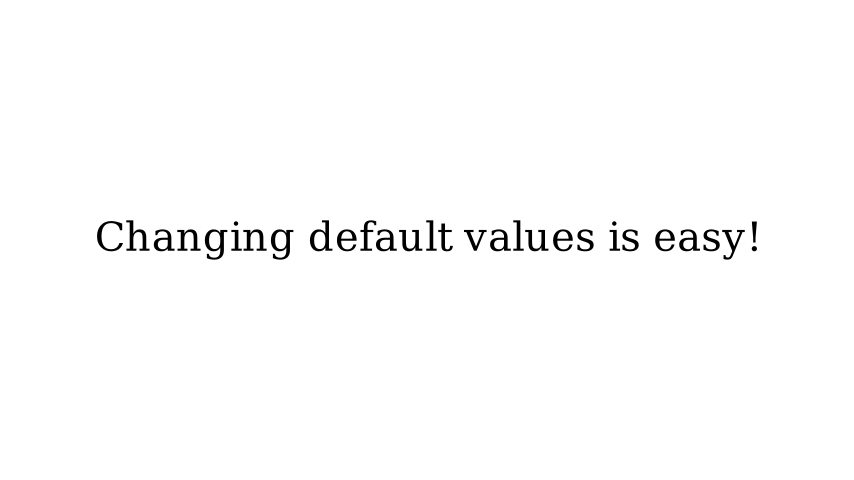from manim import *

config.background_color = WHITE

class ChangedDefaultTextcolor(Scene):
def construct(self):
Text.set_default(color=BLACK)

# we revert the colour back to the default to prevent a bug in the docs.
Text.set_default(color=WHITE)

set_x(x, direction=array([0., 0., 0.]))[source]#

Set x value of the center of the Mobject (int or float)

set_y(y, direction=array([0., 0., 0.]))[source]#

Set y value of the center of the Mobject (int or float)

set_z(z, direction=array([0., 0., 0.]))[source]#

Set z value of the center of the Mobject (int or float)

set_z_index(z_index_value, family=True)[source]#

Sets the Mobject’s z_index to the value specified in z_index_value.

Parameters
• z_index_value (float) – The new value of z_index set.

• family (bool) – If True, the z_index value of all submobjects is also set.

Returns

The Mobject itself, after z_index is set. For chaining purposes. (Returns self.)

Return type

Mobject

Examples

Example: SetZIndex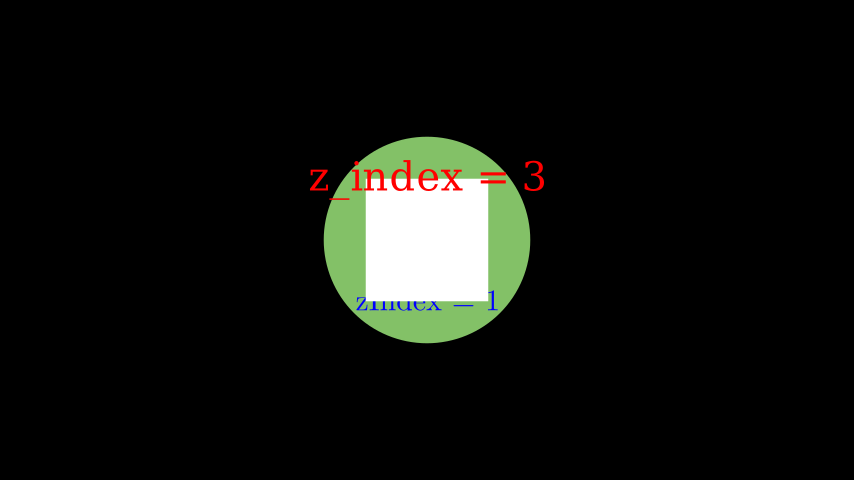from manim import *

class SetZIndex(Scene):
def construct(self):
text = Text('z_index = 3', color = PURE_RED).shift(UP).set_z_index(3)
square = Square(2, fill_opacity=1).set_z_index(2)
tex = Tex(r'zIndex = 1', color = PURE_BLUE).shift(DOWN).set_z_index(1)
circle = Circle(radius = 1.7, color = GREEN, fill_opacity = 1) # z_index = 0

# Displaying order is now defined by z_index values

set_z_index_by_z_coordinate()[source]#

Sets the Mobject’s z coordinate to the value of z_index.

Returns

The Mobject itself, after z_index is set. (Returns self.)

Return type

Mobject

shift(*vectors)[source]#

Shift by the given vectors.

Parameters

vectors (ndarray) – Vectors to shift by. If multiple vectors are given, they are added together.

Returns

self

Return type

Mobject

shuffle(recursive=False)[source]#

Shuffles the list of submobjects.

shuffle_submobjects(*args, **kwargs)[source]#

Shuffles the order of submobjects

Examples

Example: ShuffleSubmobjectsExample

from manim import *

class ShuffleSubmobjectsExample(Scene):
def construct(self):
s= VGroup(*[Dot().shift(i*0.1*RIGHT) for i in range(-20,20)])
s2= s.copy()
s2.shuffle_submobjects()
s2.shift(DOWN)
self.play(Write(s), Write(s2))

sort(point_to_num_func=<function Mobject.<lambda>>, submob_func=None)[source]#

Sorts the list of submobjects by a function defined by submob_func.

sort_submobjects(*args, **kwargs)[source]#

Sort the submobjects

stretch_to_fit_depth(depth, **kwargs)[source]#

Stretches the Mobject to fit a depth, not keeping width/height proportional.

stretch_to_fit_height(height, **kwargs)[source]#

Stretches the Mobject to fit a height, not keeping width/depth proportional.

Returns

self

Return type

Mobject

Examples

>>> from manim import *
>>> sq = Square()
>>> sq.width
2.0
>>> sq.stretch_to_fit_height(5)
Square
>>> sq.height
5.0
>>> sq.width
2.0

stretch_to_fit_width(width, **kwargs)[source]#

Stretches the Mobject to fit a width, not keeping height/depth proportional.

Returns

self

Return type

Mobject

Examples

>>> from manim import *
>>> sq = Square()
>>> sq.height
2.0
>>> sq.stretch_to_fit_width(5)
Square
>>> sq.width
5.0
>>> sq.height
2.0

suspend_updating(recursive=True)[source]#

Disable updating from updaters and animations.

Parameters

recursive (bool) – Whether to recursively suspend updating on all submobjects.

Returns

self

Return type

Mobject

update(dt=0, recursive=True)[source]#

Apply all updaters.

Does nothing if updating is suspended.

Parameters
• dt (float) – The parameter dt to pass to the update functions. Usually this is the time in seconds since the last call of update.

• recursive (bool) – Whether to recursively update all submobjects.

Returns

self

Return type

Mobject

property width#

The width of the mobject.

Return type

float

Examples

Example: WidthExample

from manim import *

class WidthExample(Scene):
def construct(self):
decimal = DecimalNumber().to_edge(UP)
rect = Rectangle(color=BLUE)
rect_copy = rect.copy().set_stroke(GRAY, opacity=0.5)